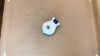Lesson 15 – Finch Fractals

Recursion

Teacher Materials

In this last lesson, you will explore recursion with the Finch. Recursion is when a function calls itself. In this lesson, you will use recursion to draw Koch fractals.Koch Fractals

The Koch fractal, also called the Koch snowflake, can be drawn with increasing levels of complexity. The level of complexity is called the order of the Koch fractal. A Koch fractal of order 0 is just a straight line with some length L. Starting from order 0, which we call the base case, we can build Koch fractals with larger orders. To create the order 1 fractal, we make four copies of the order 0 fractal; each copy has a length of L/3. We put them together so that the first and last copies lie along a straight line, while the two middle ones protrude from the line and make a 60° angle. We continue this process to make the order 2 fractal. We make four copies of the order 1 fractal where each copy is 1⁄3 the size of the original. Then we put these four copies together so that the two copies in the middle form a 60° angle. You can keep going in this way to make a fractal for any positive order n! As n approaches infinity, the length of the Koch fractal also approaches infinity because the length of each order of the fractal is 4/3 times the length of the previous order. As you zoom in on a portion of the Koch snowflake, it is self-similar, meaning that each smaller portion looks like the larger whole. The Koch snowflake is a fractal because a simple algorithm generates a complex, self-similar pattern.In the description of Koch fractals above, we started with order 0 and then worked our way up to larger orders. To draw a fractal with your Finch, you actually need to do the opposite. You start with some number n , and you want to draw a Koch fractal with that order. Luckily, there is an algorithm for this! We will use the algorithm given in How to Think Like a Computer Scientist: Learning with Python 3 by Wentworth, Elkner, Downey, and Meyers. The steps below can be used to draw a Koch fractal of order n:

• Draw Koch fractal of order n – 1 and size L/3.
• Turn left 60°.
• Draw Koch fractal of order n – 1 and size L/3.
• Turn right 120°.
• Draw Koch fractal of order n – 1 and size L/3.
• Turn left 60°.
• Draw Koch fractal of order n – 1 and size L/3.

You are going to use this algorithm to write a program that uses the Finch to draw a Koch fractal of a given order and size.

Drawing Fractals with Finch

Exercise 1

Define a function called drawFractal().

• This function should take two parameters, the order of the fractal (order) and a distance in centimeters (distance).
• Next, add the base case to your function. If the order of the fractal is 0, the robot should move forward in a straight line of length distance.
• Before moving on to the next exercise, test your function to make sure that it works as expected for order 0 (for this exercise, your robot doesn’t need to do anything for higher orders).

Exercise 2

When the order is not 0, your function will need to call itself (recursion!). If the parameters of drawFractal() are order and distance, what parameters should you use in the recursive calls to drawFractal()? Remember, you are implementing the algorithm given above Exercise 1. Fill in the blanks below.

• drawFractal( _____________ , _______________ )
• Turn left 60°
• drawFractal( _____________ , _______________ )
• Turn right 120°.
• drawFractal( _____________ , _______________ )
• Turn left 60°.
• drawFractal( _____________ , _______________ )

Exercise 3

Now implement your plan from Exercise 2. Remember, drawFractal() should only call itself when the order is greater than 0. When the order is 0, the Finch should move in a straight line. You have already implemented that part!

When testing your program, start by testing with order 1, and then work your way up to larger orders. This will make it easier to see if your program is working as you expect. Once your program is working, use a marker to draw some beautiful pictures of fractals! If you want to make a Koch Snowflake, as shown below, draw three Koch fractals with 120° turns between them.

Note: We highly recommend using a brush tip marker with the Finch. These markers work well. If you use a marker with a harder tip, the friction of the marker may make your drawing less accurate.To make more fractals with your Finch, check out this website!